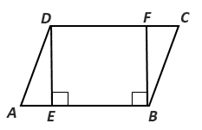Chapter 4.1, Problem 30EElementary Geometry For College St...

7th Edition
Alexander + 2 others
ISBN: 9781337614085

Solutions

Chapter
SectionElementary Geometry For College St...

7th Edition
Alexander + 2 others
ISBN: 9781337614085
Textbook Problem

In Exercises 27-30, use the definition of a parallelogram to complete each proof.Given: Parallelogram ABCD with D E - ⊥ A B - and F B -   ⊥ A B - Prove: D E - ≅ F B - Plan: First show that DEBF is a parallelogram.To determine

To prove:

DE-FB- in the given figure.

Explanation

Given:

Parallelogram ABCD with DE-AB- and FB- AB-.

Prove: DE-FB-

Corollary:

A parallelogram is a quadrilateral in which both pairs of opposite sides are parallel.

Theorem:

Two parallel line are everywhere equidistance.

From the given parallelogram ABCD, DE-AB- and FB- AB-.

In the parallelogram ABCD, by the corollary AB-DC-

Still sussing out bartleby?

Check out a sample textbook solution.

See a sample solution

The Solution to Your Study Problems

Bartleby provides explanations to thousands of textbook problems written by our experts, many with advanced degrees!

Get Started

Evaluate the expression sin Exercises 116. (32)3

Finite Mathematics and Applied Calculus (MindTap Course List)

Evaluate the integral. 33. 32xx2dx

Calculus: Early Transcendentals

In Exercises 17-20, determine whether the point lies on the graph of the function. 20. (3,113);h(t)=|t+1|t3+1

Applied Calculus for the Managerial, Life, and Social Sciences: A Brief Approach

Which vector at the right could be a gradient for f(x, y)? a b c d

Study Guide for Stewart's Multivariable Calculus, 8th

Sometimes, Always, or Never: If {an} is increasing and bounded above, then {an} converges.

Study Guide for Stewart's Single Variable Calculus: Early Transcendentals, 8th